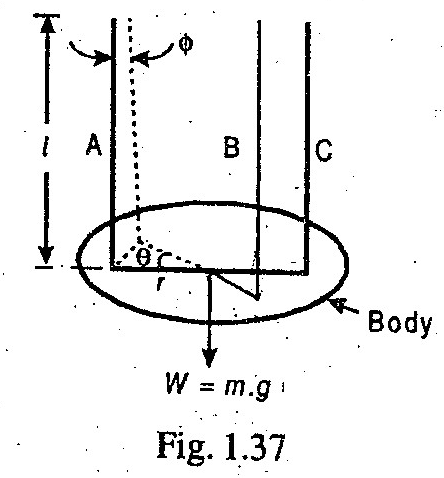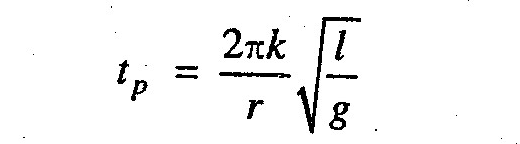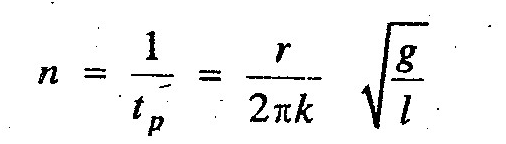### Torsional PendulumWhat is a Torsional Pendulum and formula of its periodic time? Why it is used and for what experiments?

It is used to find the moment of inertia of a body experimentally. The body (say a disc or flywheel) whose moment of inertia is to be determined is suspended by three long flexible wires A, B and C as shown in Fig. 1.37. When the body is twisted about its axis through a small angle θ and then released, it will oscillate with simple harmonic motion.

For a torsional pendulum, the periodic time is given byand frequency of oscillation,where

r = Distance of each wire from the axis of the body,
k = Radius of gyration, and
l = Length of each wire.